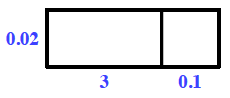Home > ACC6 > Chapter cc17 > Lesson cc17.2.2 > Problem7-56

7-56.

Calculate each of the following products without using a calculator. Homework Help ✎

1. $\frac { 7 } { 8 } \cdot \frac { 5 } { 6 }$

$\frac{a}{b}\cdot\frac{c}{d}=\frac{a \cdot\ c}{b \cdot\ d}$

1. $(3.1)(0.02)$

Use a generic rectangle, as shown below.1. $1 \frac { 3 } { 5 } \cdot 2 \frac { 1 } { 3 }$

Change the mixed numbers into fractions and then refer to part (a) for help.

1. $\frac { 2 } { 3 } \cdot \frac { 20 } { 7 }$

$\frac{40}{21}=1\frac{19}{21}$